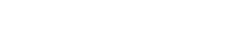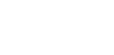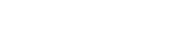i to the i-th power

Ever wondered what the imaginary unit i raised to itself is equal to? Is it going to be a complex number? Or a real one? Let’s find out! Let’s first define i: Therefore, There is an identity in maths which is in my opinion one of the most beautiful equations that exist, and that is Euler’s identity: This means At this point we have two expressions: We can use the second one to replace the -1 in the first: We no longer have the i because i times i equals… Read more i to the i-th powerHard looking yet beautiful integral

Never judge a book by its cover: this is the case. This integral requires a bit of work and knowledge, as you need to know about the Gaussian integral (I… Read more Hard looking yet beautiful integralGaussian integral using Feynman’s technique

In my last post we evaluated the following definite integral This is the formula we got: and this is the integral we want to evaluate: which is equivalent to because… Read more Gaussian integral using Feynman’s techniqueIntegral of e^-x^t using Feynman’s technique

Wouldn’t it be nice to generalize the Gaussian integral to any exponent of –x? This is the famous integral: Since this is an even function, it can be written as… Read more Integral of e^-x^t using Feynman’s techniqueArc length formula

Today we are going to find the formula that allows us to calculate the arc length given a function f(x). This is quite intuitive, so you should have no problem!… Read more Arc length formulaIntegral of sin(x)/x from 0 to infinity | A satisfying solution

Today we have a tough integral: not only is this a special integral (the sine integral Si(x)), but it also goes from 0 to infinity! Because of the first characteristic,… Read more Integral of sin(x)/x from 0 to infinity | A satisfying solutionIntegral of e^(ax)*sin(x)

In this post we are going to integrate this seemingly hard integral, which in my opinion is actually very interesting! When we have a product of a trigonometric and a… Read more Integral of e^(ax)*sin(x)Integral of sec²(x)/exp(tan(x))

Although it may look a bit intimidating at first, I can guarantee you this is actually a very easy integral! But what integration technique do you think will be useful?… Read more Integral of sec²(x)/exp(tan(x))

{{#pages}} {{/pages}}
%%footer%%